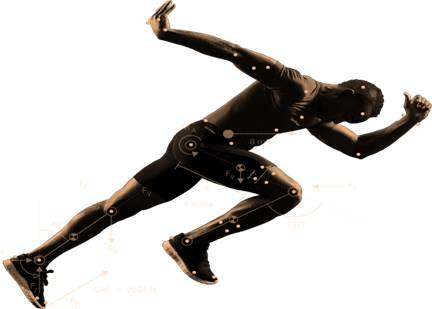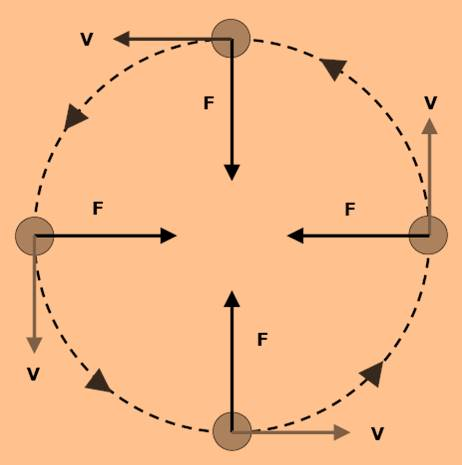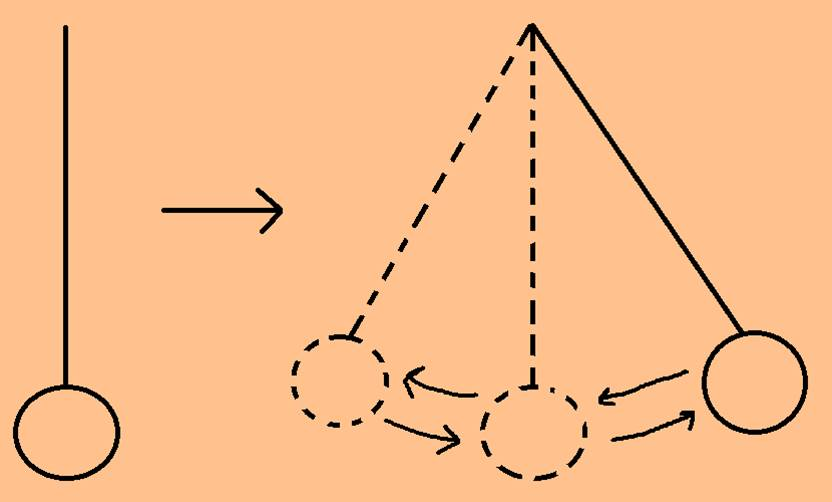Register for Maths, Science, English, Reasoning Olympiad Exams Register here | Book Free Demo for Live Olympiad Classes here| Check Olympiad Exam Dates here | Buy Practice Papers for IMO, IOM, HEO, IOEL etc here | Login here to participate in all India free mock test on every Saturday

Motion And TimeMotion

With elapse of time when the position of an object changes to some stationary object like an electric pole, building, then that object is said to be in motion.Fast and slow motion

It depends on the distance covered in a time. The object covering a distance in less time becomes fast moving object and an object covering a distance in less time becomes a slow moving object.

Rectilinear motion

When a motion is along a straight line then it is called a motion in a straight line or rectilinear motion.

Curvilinear motion

When an object moves along a curve line then it is called as curvilinear motion.

Circular motion

When the motion is around a circle, then it is called a circular motion. For example – A cyclist moving around the park.Speed

In a particular time distance covered by an object is called as speed.

Distance = Speed*Time

Uniform speed

When the distance covered in each number of units is fixed then that motion is said to be uniform speed or uniform motion.

Non-Uniform speed

When the distance covered in each number of units is not fixed then that motion is said to be Non-uniform speed or Non-uniform motion.

Oscillatory motion

When after each fixed interval of time, the motion of the object repeats then the motion is called as oscillatory motion or periodic motion.

A pendulum is an example of such motion. One oscillation is completed when a pendulum starts from one end and comes back to the origin.

Time period means the time taken by the pendulum to complete one oscillation.Unit of time

Seconds is the unit to measure time and is denoted by ‘S’.

• 60 seconds = 1 minute
• 60 minutes = 1 hour
• 24 hours = 1 day
• 7 days = 1 week
• 365 weeks = 1 year

Unit of speed

Meter/minute (m/min) or meter/second (m/s) is the unit of speed.

The speed of a vehicle is calculated by a device called speedometer.

Recap

• With elapse of time when the position of an object changes to some stationary object like an electric pole, building, then that object is said to be in motion.
• There are two types of motion-

- Slow motion

- Fast motion

• When an object moves along a curve line then it is called as curvilinear motion.
• In a particular time distance covered by an object is called as speed.
• When after each fixed interval of time, the motion of the object repeats then the motion is called as oscillatory motion or periodic motion.
• Seconds is the unit to measure time and is denoted by ‘S’.
• Meter/minute (m/min) or meter/second (m/s) is the unit of speed.